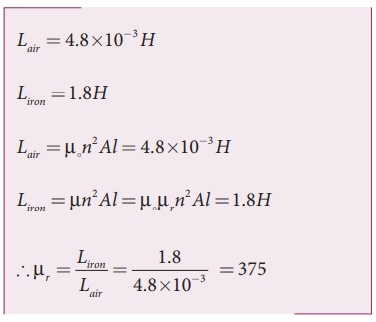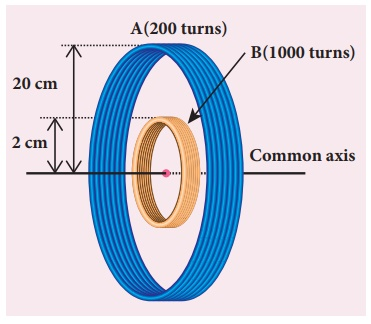Home | | Physics 12th Std | Eddy Currents, Self-inductance, Mutual inductance: Solved Example Problems

# Eddy Currents, Self-inductance, Mutual inductance: Solved Example Problems

Physics : Electromagnetic Induction: Eddy Currents, Self-inductance, Mutual inductance: Solved Example Problems with Answers, Solution and Explanation

## Self-inductance of a long solenoid(Eddy Currents): Solved Example Problems

EXAMPLE 4.10

A solenoid of 500 turns is wound on an iron core of relative permeability 800. The length and radius of the solenoid are 40 cm and 3 cm respectively. Calculate the average emf induced in the solenoid if the current in it changes from 0 to 3 A in 0.4 second.

Solution

N = 500 turns; ┬Ąr = 800 ;

l = 40 cm = 0.4 m; r = 3 cm = 0.03 m;

di = 3 ŌĆō 0 = 3 A; dt = 0.4 s

Self inductance,EXAMPLE 4.11

The self-inductance of an air-core solenoid is 4.8 mH. If its core is replaced by iron core, then its self-inductance becomes 1.8 H. Find out the relative permeability of iron.

Solution## Mutual inductance between two long co-axial solenoids(Eddy Curent): Solved Example Problems

EXAMPLE 4.12

The current flowing in the first coil changes from 2 A to 10 A in 0.4 sec. Find the mutual inductance between two coils if an emf of 60 mV is induced in the second coil. Also determine the induced emf in the second coil if the current in the first coil is changed from 4 A to 16 A in 0.03 sec. Consider only the magnitude of induced emf.

Solution

Case (i):

di1 = 10 ŌĆō 2 = 8 A; dt = 0.4 s;

╬Ą2 = 60 ├Ś10ŌłÆ3V

Case (ii):

di1 = 16 ŌĆō 4 = 12 A;

dt = 0.03 s

(i) Mutual inductance of the second coil with respect to the first coil(ii) Induced emf in the second coil due to the rate of change of current in the first coil isEXAMPLE 4.13

Consider two coplanar, co-axial circular coils A and B as shown in figure. The radius of coil A is 20 cm while that of coil B is 2 cm. The number of turns is 200 and 1000 for coils A and B respectively. Calculate the mutual inductance of coil B with respect to coil A. If the current in coil A changes from 2 A to 6 A in 0.04 sec, determine the induced emf in coil B and the rate of change of flux through the coil B at that instant.Solution

NA = 200 turns; NB = 1000 turns;

rA = 20 ├Ś 10-2 m; rB = 2 ├Ś 10-2 m;

dt = 0.04 s; diA = 6ŌłÆ2 = 4A

Let iA be the current flowing in coil A, then the magnetic field BA at the centre of the circular coil A isThe rate of change of magnetic flux of coil isStudy Material, Lecturing Notes, Assignment, Reference, Wiki description explanation, brief detail
12th Physics : Electromagnetic Induction and Alternating Current : Eddy Currents, Self-inductance, Mutual inductance: Solved Example Problems |